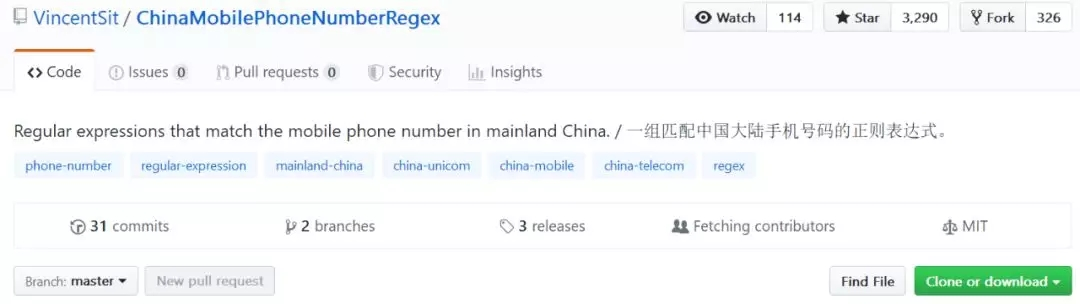# 分享一组开源的匹配中国大陆手机号码的正则表达式

更新时间：2019年06月21日 14:14:47   作者：程序猿

• 比如判断一个手机号的格式是否正确
• 手机号码的匹配
• 填写的邮箱格式是否正确

^(?:+?86)?1(?:3d{3}|5[^4D]d{2}|8d{3}|7(?:d{2}|4(?:0d|1[0-2]|9d))|9d{2}|6d{2}|4(?:0d{3}|d{4}|d{2}))d{6}\$

^(?:+?86)?1(?:3d{3}|5[^4D]d{2}|8d{3}|7(?:d{2}|4(?:0d|1[0-2]|9d))|9d{2}|6d{2}|4d{2})d{6}\$

^(?:+?86)?1(?:3d{3}|5[^4D]d{2}|8d{3}|7(?:d{2}|4(?:0d|1[0-2]|9d))|9d{2}|66d{2})d{6}\$

^(?:+?86)?1(?:3(?:4[^9D]|[5-9]d)|5[^3-6D]d|8d|(?:78|98)d)d{7}\$

^(?:+?86)?1(?:3[0-2]||66)d{8}\$

^(?:+?86)?1(?:3(?:3d|49)d|53d{2}|8d{2}|7(?:d{2}|40[0-5])|9d{2})d{6}\$

^(?:+?86)?1749d{7}\$

^(?:+?86)?174(?:0[6-9]|1[0-2])d{6}\$

^(?:+?86)?1(?:7|6)d{8}\$

^(?:+?86)?1(?:65d|70)d{7}\$

^(?:+?86)?1(?:70|71d|67d)d{7}\$

^(?:+?86)?170[0-2]d{7}\$

^(?:+?86)?14(?:0|d)d{9}\$

^(?:+?86)?14(?:40|8d)d{9}\$

^(?:+?86)?146d{10}\$

^(?:+?86)?1410d{9}\$

^(?:+?86)?14d{8}\$

^(?:+?86)?147d{8}\$

^(?:+?86)?145d{8}\$

^(?:+?86)?149d{8}\$